# Trapezoid

Here you will learn about a trapezoid, including the properties of a trapezoid, how to identify a trapezoid, and how to classify a trapezoid.

Students will first learn about the trapezoid as part of geometry in 1 st grade, but they will learn about the properties of a trapezoid in 5 th grade.

## What is a trapezoid?

A trapezoid is a type of quadrilateral, which is a polygon with four straight sides. A trapezoid has one pair of parallel sides which are called the bases of the trapezoid. The lengths of the bases are not congruent. The other two sides of the trapezoid are called the legs.

The legs may be different lengths, but they are not parallel to each other. The angles that share the same base are called base angles.

Properties of a trapezoid

In order for a polygon to be a trapezoid, it must have the following properties:

Four sides: A trapezoid is a four-sided polygon.

Two parallel sides: A trapezoid has two sides that are parallel to each other. These are called the “bases.”

Two non-parallel sides: The other two sides are not parallel to each other. These are often referred to as the “legs.”

Opposite angles: The angles formed by the longer base and each leg are equal to each other (congruent). The same goes for the angles formed by the shorter base and each leg.

Diagonals: A trapezoid has two diagonals, which are line segments connecting non-adjacent vertices. These diagonals are not equal in length.

There are three types of trapezoids:

Isosceles trapezoid
Scalene trapezoid
Right trapezoid

An isosceles trapezoid has a pair
of non-parallel sides that are
equal in length and two pairs of
congruent angles.

A scalene trapezoid has no
congruent angles or side lengths.

A right trapezoid has one
pair of right angles.

Classification of a trapezoid

As you can see in the quadrilateral hierarchy, a trapezoid classifies as a quadrilateral because it has 4 sides. It does not classify as a parallelogram like a rectangle, rhombus, or square because, unlike those shapes, a trapezoid only has 1 pair of parallel sides instead of 2.

### What is a trapezoid?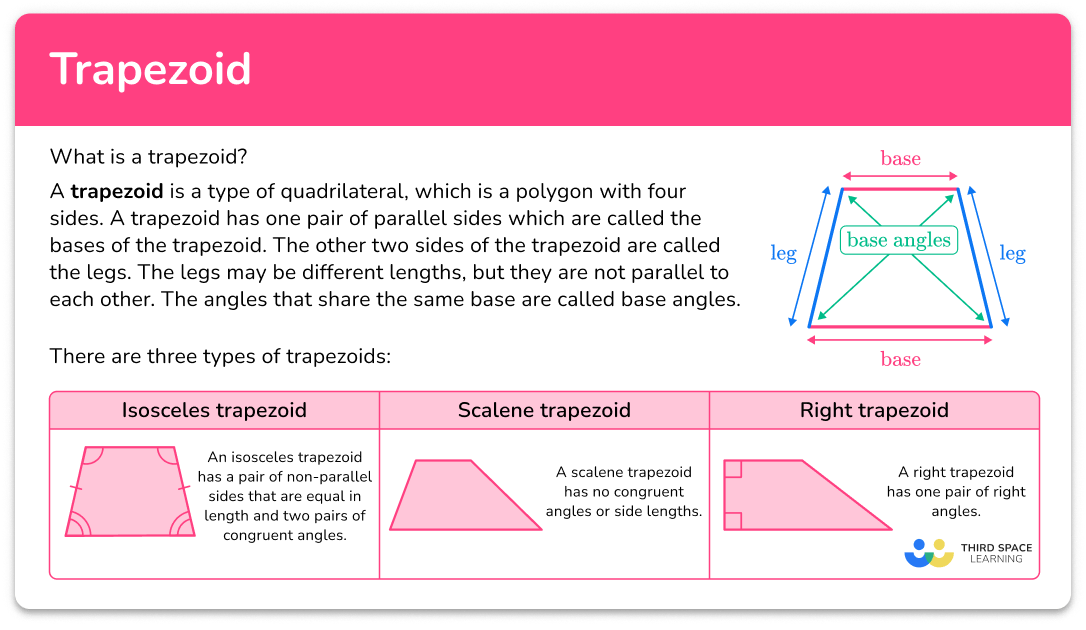## Common Core State Standards

How does this relate to 5 th grade math?

• Grade 5 – Geometry (5.G.B.3)
Understand that attributes belonging to a category of two-dimensional figures also belong to all subcategories of that category. For example, all rectangles have four right angles and squares are rectangles, so all squares have four right angles.

• Grade 5 – Geometry (5.G.B.4)
Classify two-dimensional figures in a hierarchy based on properties.

## How to identify a trapezoid

In order to identify a trapezoid:

1. Look for the characteristics of a trapezoid.
2. State whether or not the shape is a trapezoid.
3. If the shape is not a trapezoid, explain what characteristics are different.

## Trapezoid examples

### Example 1: identify trapezoids

Is this shape a trapezoid?

1. Look for the characteristics of a trapezoid.

For a shape to be a trapezoid, it must have 4 sides and 1 pair of parallel sides.

2State whether or not the shape is a trapezoid.

Since this shape has 4 sides and 1 pair of parallel sides, it is a trapezoid.

### Example 2: identify trapezoids

Is this shape a trapezoid?

For a shape to be a trapezoid, it must have 4 sides and 1 pair of parallel sides.

This shape is not a trapezoid.

Since this shape has 4 sides but no pairs of parallel sides, it is NOT a trapezoid.

### Example 3: identify types of trapezoids

Is this shape a trapezoid? If so, what type of trapezoid is it?

For a shape to be a trapezoid, it must have 4 sides and 1 pair of parallel sides.

This shape is a trapezoid. Since it has one pair of right angles, it is a right trapezoid.

### Example 4: identify types of trapezoids

Which trapezoid is a scalene trapezoid?

For a shape to be a trapezoid, it must have 4 sides and 1 pair of parallel sides. For a trapezoid to be a scalene trapezoid, it must also have no congruent side lengths or angles.

The 3 rd trapezoid is a scalene trapezoid.

The first two trapezoids are isosceles trapezoids since they have a pair of opposite sides that are congruent and two pairs of congruent angles. The last trapezoid is a right trapezoid since it has a pair of right angles.

### Example 5: identify types of a trapezoid

Which trapezoid is an isosceles trapezoid?

For a shape to be a trapezoid, it must have 4 sides and 1 pair of parallel sides. For a trapezoid to be an isosceles trapezoid, it must also have a pair of opposite sides that are congruent and two pairs of congruent angles.

The 2 nd trapezoid is an isosceles trapezoid.

The first trapezoid is a scalene trapezoid since it has no congruent side lengths or angles. The last trapezoid is a right trapezoid since it has a pair of right angles.

### Example 6: trapezoid classification

Marnie says this shape is a trapezoid, a quadrilateral, and a parallelogram. Is she correct?

For a shape to be a trapezoid, it must have 4 sides and 1 pair of parallel sides.

This shape is a trapezoid.

This shape is a trapezoid. It is also a quadrilateral because it has 4 sides. It is not a parallelogram because a trapezoid only has 1 pair of parallel sides and a parallelogram has 2. Therefore, Marnie is incorrect.

### Teaching tips for trapezoids

• Provide real-life examples of trapezoids to help students connect the concept to their surroundings. For instance, point out trapezoidal shapes in buildings, road signs, or furniture.

• Discuss the properties of a trapezoid. Highlight that the non-parallel sides of a trapezoid are the legs, while the parallel sides are the bases of the trapezoid. Emphasize that the bases have to be different lengths, so there is always a longer base.

For example,

If the bases are congruent,
there are two ways a quadrilateral
can be formed
1. The bases are directly over
each other: the legs are also
parallel and form right angles.

Notice this forms a rectangle,
which is NOT a trapezoid.
2. The bases are not
directly over each other:
the legs are also parallel.

Notice this forms a parallelogram,
which is NOT a trapezoid.

• Present students with worksheets that include problem-solving tasks involving trapezoids. For example, ask them to calculate the perimeter of a trapezoid or the area of a trapezoid using given measurements. Encourage them to explain their reasoning and share their solutions with the class.

### Easy mistakes to make

• Thinking that a trapezoid has at least one pair of parallel lines instead of exactly one pair
Some students may think any shape with at least one pair of parallel lines can be classified as a trapezoid, but this is incorrect. To be a trapezoid, a shape must have exactly one pair of parallel lines.

• Incorrectly identifying parallel sides
Identifying the parallel sides of a trapezoid can be challenging for some students. They may mistakenly choose non-parallel sides as the bases of the trapezoid. Reinforce the definition of a trapezoid as a quadrilateral with one pair of parallel sides. Encourage students to look for the sides that are always the same distance apart and never intersect when extended.

### Practice trapezoid questions

1. Which shape is a trapezoid?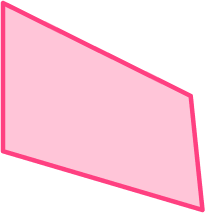The last shape is the only shape that has 4 sides and exactly 1 pair of parallel sides.

2. Which shape is not a trapezoid?The first shape is not a trapezoid. It has 4 sides, but it does not have a pair of parallel sides.

3. What are the properties of a trapezoid?

4 sides, 2 pairs of parallel sides4 sides, 2 pairs of parallel sides, 2  right angles4 sides, 1 pair of parallel sides4 sides, 1 pair of parallel sides, 4 right anglesTo be a trapezoid, a shape must have 4 sides and 1 pair of parallel sides.

4. A trapezoid is also a …

parallelogram3D shapetriangleSince a trapezoid has 4 sides, it classifies as a quadrilateral.

5. Name the type of trapezoid.scalene trapezoidisosceles trapezoidright trapezoidequilateral trapezoidIn addition to 4 sides and 1 pair of parallel sides, an isosceles trapezoid must also have a pair of opposite sides that are congruent and two pairs of congruent angles.

6. Which trapezoid is a right trapezoid?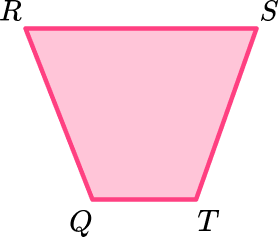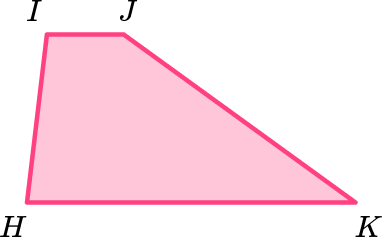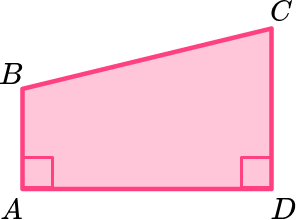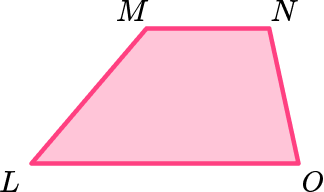In addition to 4 sides and 1 pair of parallel sides, a right trapezoid must also have a pair of right angles. Trapezoid ABCD is a right trapezoid.

## Trapezoid FAQs

What is the definition of trapezoids?

Trapezoids are quadrilaterals ( 4 -sided shape) with 1 pair of parallel sides.

What are the properties of a trapezoid?

A trapezoid has 4 sides and 1 pair of parallel sides.

How do you classify a trapezoid?

A trapezoid can be classified as a polygon and a quadrilateral.

How do you find the area of a trapezoid?

To find the area, you can use the area of a trapezoid formula which is
A = \cfrac{1}{2} \, (a + b) \, h. Students likely won’t be asked to find the area of the trapezoid until middle school or high school.

What is the median of a trapezoid?

The median of a trapezoid is a line segment that connects the midpoints of the two non-parallel sides of a trapezoid.

What is the difference between a trapezoid and a trapezium?

A trapezoid and a trapezium are one and the same; other English-speaking countries, such as the UK, refer to a trapezoid as a trapezium.

## Still stuck?

At Third Space Learning, we specialize in helping teachers and school leaders to provide personalized math support for more of their students through high-quality, online one-on-one math tutoring delivered by subject experts.

Each week, our tutors support thousands of students who are at risk of not meeting their grade-level expectations, and help accelerate their progress and boost their confidence.